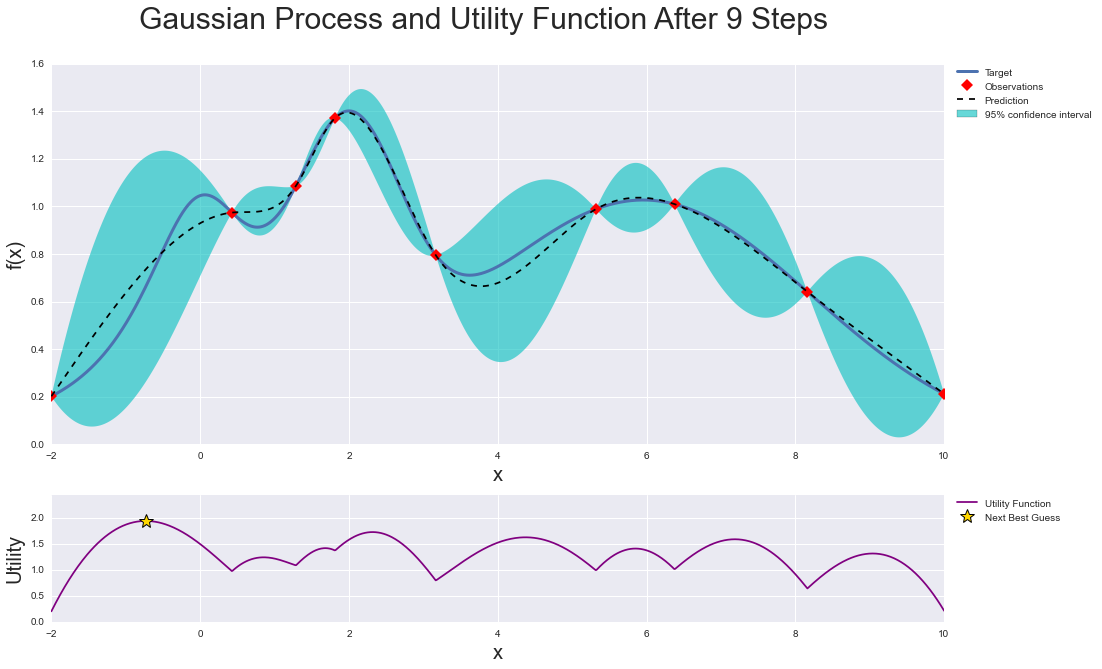# Plotting confidence intervals between points on a graph

I was actually doing some work regarding Bayesian Optimization and came across this graph.

I know this may appear to be a very naive question but.... what is the exact name of this graph (x-f(x) plot) given below that contains the confidence intervals between the points? I am asking about the interval/bound part (the blue region between red points) and how is this confidence bound computed between any two adjacent points.